# Maharashtra Board 11th Commerce Maths Solutions Chapter 8 Linear Inequations Ex 8.3

Balbharati Maharashtra State Board 11th Commerce Maths Solution Book Pdf Chapter 8 Linear Inequations Ex 8.3 Questions and Answers.

## Maharashtra State Board 11th Commerce Maths Solutions Chapter 8 Linear Inequations Ex 8.3

Find the graphical solution for the following system of linear inequations.

Question 1.
x – y ≤ 0, 2x – y ≥ -2
Solution:
To find a graphical solution, construct the table as follows: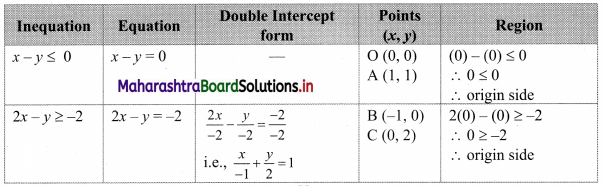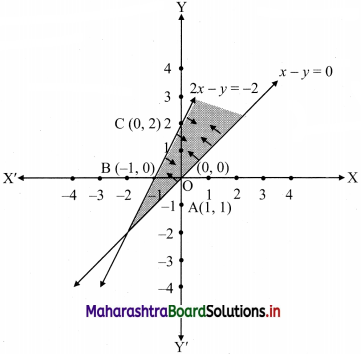The shaded portion represents the graphical solution.

Question 2.
2x + 3y ≥ 12, -x + y ≤ 3, x ≤ 4, y ≥ 3
Solution:
To find a graphical solution, construct the table as follows: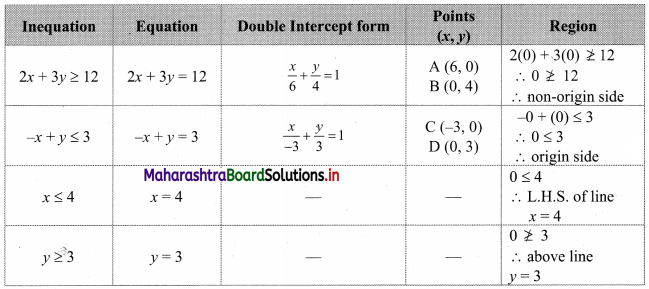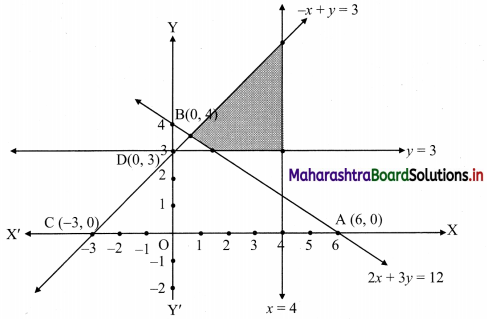The shaded portion represents the graphical solution.Question 3.
3x + 2y ≤ 1800, 2x + 7y ≤ 1400
Solution:
To find a graphical solution, construct the table as follows: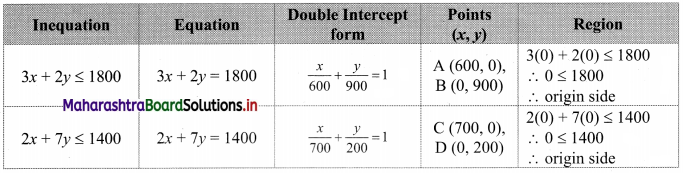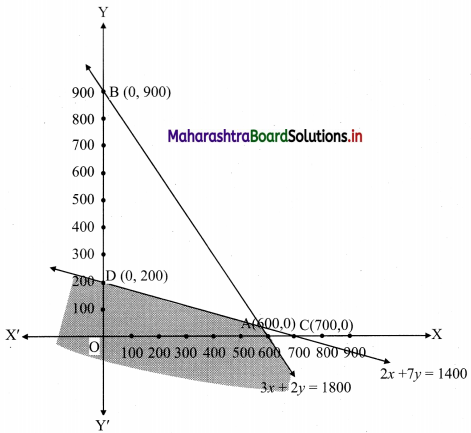The shaded portion represents the graphical solution.

Question 4.
0 ≤ x ≤ 350, 0 ≤ y ≤ 150
Solution:
To find a graphical solution, construct the table as follows: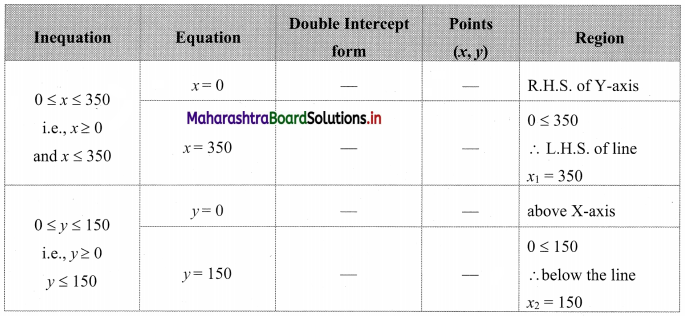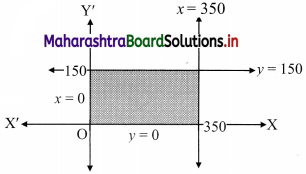The shaded portion represents the graphical solution.

Question 5.
$$\frac{x}{60}+\frac{y}{90}$$ ≤ 1, $$\frac{x}{120}+\frac{y}{75}$$ ≤ 1, x ≥ 0, y ≥ 0
Solution:
To find a graphical solution, construct the table as follows: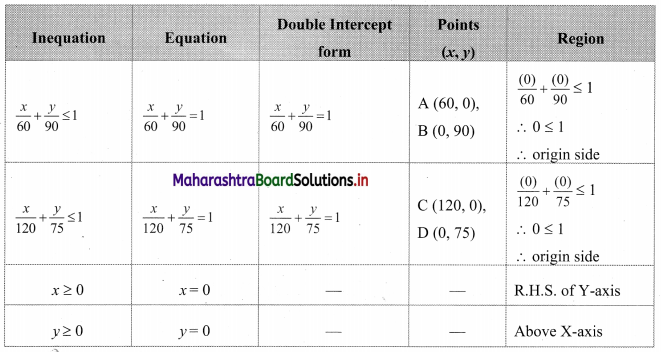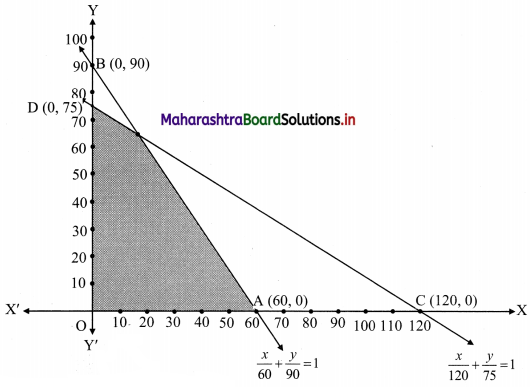The shaded portion represents the graphical solution.Question 6.
3x + 2y ≤ 24, 3x + y ≥ 15, x ≥ 4
Solution:
To find a graphical solution, construct the table as follows: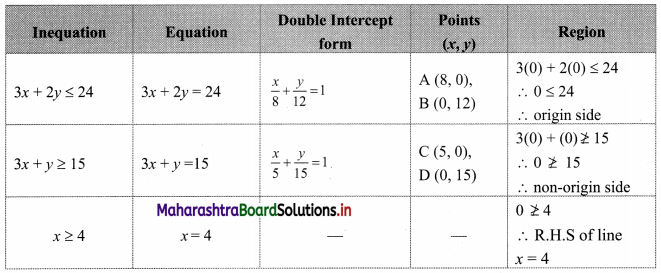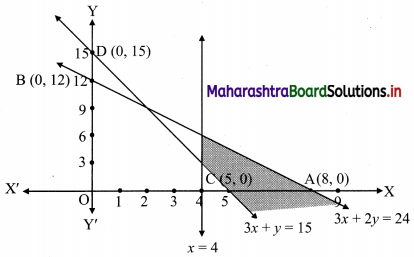The shaded portion represents the graphical solution.

Question 7.
2x + y ≥ 8, x + 2y ≥ 10, x ≥ 0, y ≥ 0
Solution:
To find a graphical solution, construct the table as follows: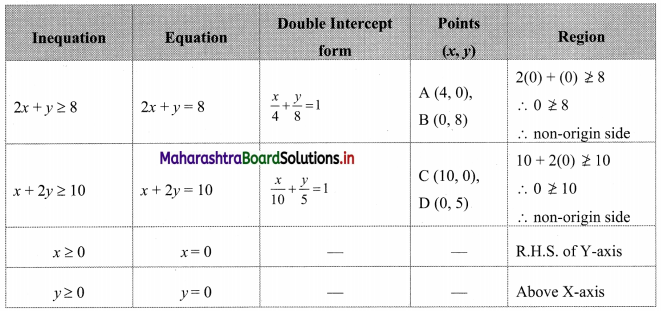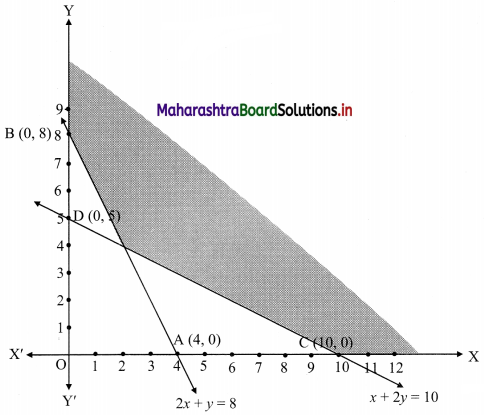The shaded portion represents the graphical solution.